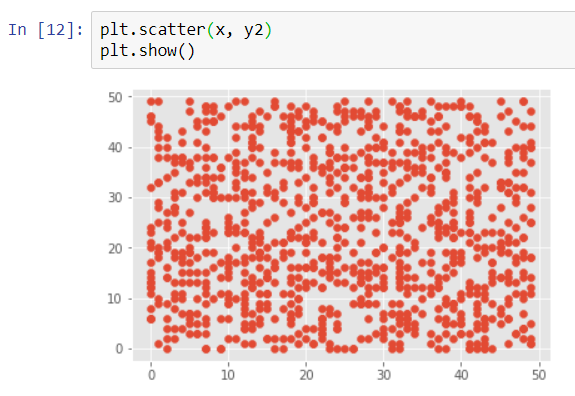### What is Redundancy?

Let us suppose that we have a dataset where there are 10 attributes. Now suppose that out of these 10, there is an attribute that can be derived or calculated from some of the other attributes. Such attributes that can be derived from other attributes are known as Redundant attributes.

### How do we find a Redundant attribute?

We can check if an attribute is redundant or not using correlation analysis. Given two attributes, we can measure how strongly one attribute implies the other, based on the available data.

Let us suppose we have numeric attributes. We can use the correlation coefficient and covariance, both of which assess how one attribute’s values vary from those of another.

#### Correlation Coefficient for Numerical Data

For numerical data, we can calculate the correlation coefficient (also known as Pearson’s product moment coefficient) for calculating the correlation between the attributes.

The formula for correlation coefficient goes as -There are a few points to be notes based on the above formula -

• Correlation values range from (-1,1).
• The attributes are said to be highly correlated if the correlation value is closer to -1 or 1.
• 0 means no correlation. If the correlation value is close to 0, it is known as a weak correlation.
• If the correlation value is positive, it is said to be a regular correlation.
• If the correlation value is negative, it is said to be an inverse correlation.

Let us now examine our understanding of correlation in python, using Pandas and NumPy.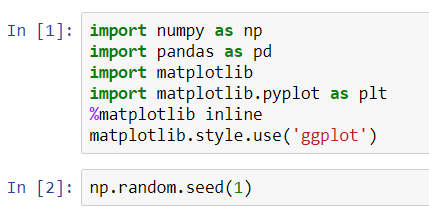Let us first create our first attribute.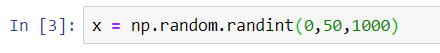Here, x is a list of 1000 integers ranging from 0 to 50.

Let us create another attribute against which we are going to measure the correlation values.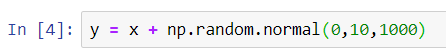We can see from the above equation that y is sincerely correlated with x. Let us calculate the correlation value and then visualize this using matplotlib.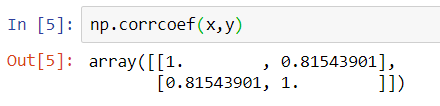NumPy gives us a function corrcoef() to calculate the correlation value between two attributes.

Here, the correlation value is 0.815, which is nearly close to 1. So we can say that the two attributes are highly correlated.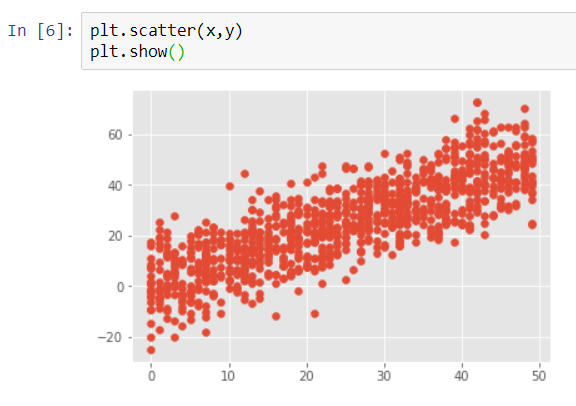The above scatter plot shows that there is a Linear Positive Correlation between x and y. Hence, x and y are highly correlated.

Let us create another attribute to understand Negative Correlation.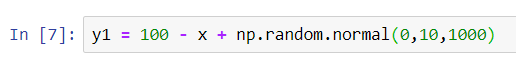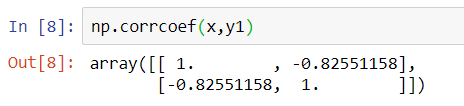A correlation value of -0.8255 signifies a strong negative correlation. Let us visualize this using matplotlib.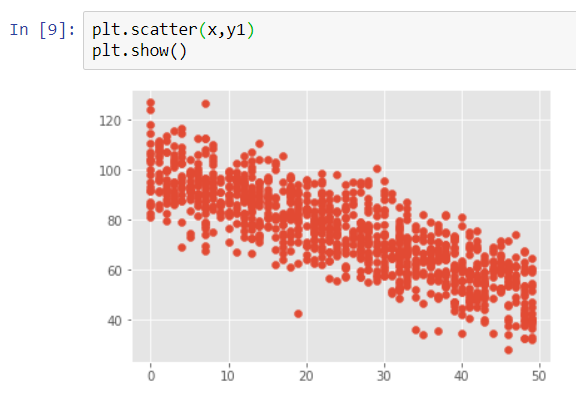Let us create just one more last attribute to understand zero or weak correlation between two attributes.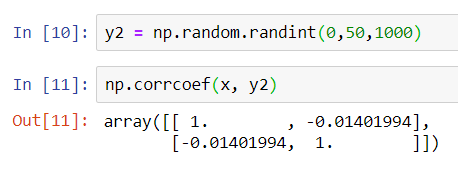A correlation value of -0.014 is nearly close to 0. This tells us the correlation between the two attributes is weak. Let us visualize this too using matplotlib.